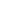If a+b =12 , ab =22, then (a2 + b2) is equal to:
a) 188
b) 144
c) 64
d) 100
SSC CHSL-2014

 100. Solution Squaring a+b; (a+b)2 = a2+ b2 + 2ab 144 = a2+ b2 + 44
Share :
+
Next
« Prev
Prev
Next »